Test: Systems of Linear Equations, Matrix Algebra & Transform Theory- 1

# Test: Systems of Linear Equations, Matrix Algebra & Transform Theory- 1

Test Description

## 20 Questions MCQ Test GATE Mechanical (ME) 2023 Mock Test Series | Test: Systems of Linear Equations, Matrix Algebra & Transform Theory- 1

Test: Systems of Linear Equations, Matrix Algebra & Transform Theory- 1 for Civil Engineering (CE) 2023 is part of GATE Mechanical (ME) 2023 Mock Test Series preparation. The Test: Systems of Linear Equations, Matrix Algebra & Transform Theory- 1 questions and answers have been prepared according to the Civil Engineering (CE) exam syllabus.The Test: Systems of Linear Equations, Matrix Algebra & Transform Theory- 1 MCQs are made for Civil Engineering (CE) 2023 Exam. Find important definitions, questions, notes, meanings, examples, exercises, MCQs and online tests for Test: Systems of Linear Equations, Matrix Algebra & Transform Theory- 1 below.
Solutions of Test: Systems of Linear Equations, Matrix Algebra & Transform Theory- 1 questions in English are available as part of our GATE Mechanical (ME) 2023 Mock Test Series for Civil Engineering (CE) & Test: Systems of Linear Equations, Matrix Algebra & Transform Theory- 1 solutions in Hindi for GATE Mechanical (ME) 2023 Mock Test Series course. Download more important topics, notes, lectures and mock test series for Civil Engineering (CE) Exam by signing up for free. Attempt Test: Systems of Linear Equations, Matrix Algebra & Transform Theory- 1 | 20 questions in 60 minutes | Mock test for Civil Engineering (CE) preparation | Free important questions MCQ to study GATE Mechanical (ME) 2023 Mock Test Series for Civil Engineering (CE) Exam | Download free PDF with solutions
 1 Crore+ students have signed up on EduRev. Have you?
Test: Systems of Linear Equations, Matrix Algebra & Transform Theory- 1 - Question 1

### The system of linear equations 4x + 2y = 7 2x + y = 6              has

Detailed Solution for Test: Systems of Linear Equations, Matrix Algebra & Transform Theory- 1 - Question 1

(b) This can be written as AX = B Where A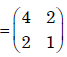Angemented matrix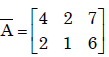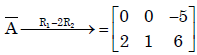rank(A) ≠ rank(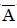). The system is inconsistant .So system has no solution.

Test: Systems of Linear Equations, Matrix Algebra & Transform Theory- 1 - Question 2

### For the following set of simultaneous equations: 1.5x – 0.5y = 2 4x + 2y + 3z = 9 7x + y + 5z = 10

Detailed Solution for Test: Systems of Linear Equations, Matrix Algebra & Transform Theory- 1 - Question 2

(a)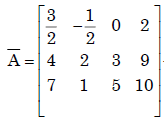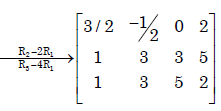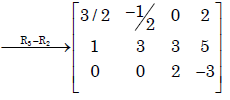∴ rank of() = rank of(A) = 3

∴ The system has unique solution.

Test: Systems of Linear Equations, Matrix Algebra & Transform Theory- 1 - Question 3

### The following set of equations has 3 x + 2 y + z = 4 x – y + z = 2 ​-2 x + 2 z = 5

Detailed Solution for Test: Systems of Linear Equations, Matrix Algebra & Transform Theory- 1 - Question 3

(b)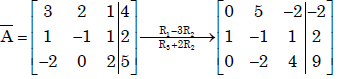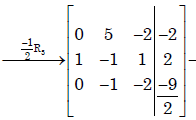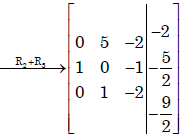∴ rank (A) = rank () = 3
∴ The system has unique solution

Test: Systems of Linear Equations, Matrix Algebra & Transform Theory- 1 - Question 4

Consider the system of simultaneous equations
x + 2y + z = 6
2x + y + 2z =  6
x + y +  z = 5
This system has

Detailed Solution for Test: Systems of Linear Equations, Matrix Algebra & Transform Theory- 1 - Question 4

(c )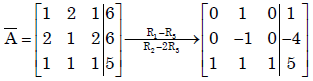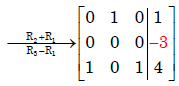∴ rank(A) = 2 ≠ 3 = rank() .

∴ The system is inconsistent and has no solution.

Test: Systems of Linear Equations, Matrix Algebra & Transform Theory- 1 - Question 5

Multiplication of matrices E and F is G. Matrices E and G are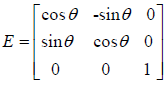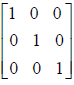What is the matrix F?

Detailed Solution for Test: Systems of Linear Equations, Matrix Algebra & Transform Theory- 1 - Question 5

(c)
Given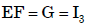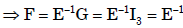Test: Systems of Linear Equations, Matrix Algebra & Transform Theory- 1 - Question 6

Consider a non-homogeneous system of linear equations representing mathematically an over-determined system. Such a system will be

Detailed Solution for Test: Systems of Linear Equations, Matrix Algebra & Transform Theory- 1 - Question 6

Ans.(b)
In an over determined system having more equations than variables, it is necessary to have consistent having many solutions .

Test: Systems of Linear Equations, Matrix Algebra & Transform Theory- 1 - Question 7

For the set of equations
x1 + 2x  + x3 + 4x4 = 0
3x1 + 6x2 + 3x3 + 12x4 = 0

Detailed Solution for Test: Systems of Linear Equations, Matrix Algebra & Transform Theory- 1 - Question 7

given set of equations are x1+2x2+x3+4x4=2 , 3x1+6x2+3x3+12x4=6

consider AB =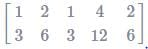⇒ R2 → R2 - 3R1

AB =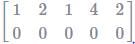P(A)=1; P(AB)=1;n=4

⇒ P(A) =P(B) < no. of variables

⇒ Infinitely many solutions ⇒multiple non-trivial solution

Test: Systems of Linear Equations, Matrix Algebra & Transform Theory- 1 - Question 8

Let P ≠ 0 be a 3 × 3 real matrix. There exist linearly independent vectors x and y such that Px = 0 and Py = 0. The dimension of the range space of P is

Detailed Solution for Test: Systems of Linear Equations, Matrix Algebra & Transform Theory- 1 - Question 8

(b)

Test: Systems of Linear Equations, Matrix Algebra & Transform Theory- 1 - Question 9

The eigen values of a skew-symmetric matrix are

Detailed Solution for Test: Systems of Linear Equations, Matrix Algebra & Transform Theory- 1 - Question 9

(c)

Test: Systems of Linear Equations, Matrix Algebra & Transform Theory- 1 - Question 10

The rank of a 3×3 matrix C (=AB), found by multiplying a non-zero column matrix Aof size 3×1 and a non-zero row matrix B of size 1×3, is

Detailed Solution for Test: Systems of Linear Equations, Matrix Algebra & Transform Theory- 1 - Question 10

(b)

Let A =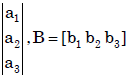Then C = AB =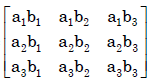Then det (AB) = 0.

Then also every minor
of order 2 is also zero.
∴ rank(C) =1.

Test: Systems of Linear Equations, Matrix Algebra & Transform Theory- 1 - Question 11

Match the items in columns I and II.
​Column I                                               Column II
P. Singular matrix                               1. Determinant is not defined
Q. Non-square matrix                          2. Determinant is always one
R. Real symmetric                              3. Determinant is zero
S. Orthogonal matrix                           4. Eigenvalues are always real
5. Eigenvalues are not defined

Detailed Solution for Test: Systems of Linear Equations, Matrix Algebra & Transform Theory- 1 - Question 11

(a) (P) Singular matrix → Determinant is zero
(Q) Non-square matrix → Determinant is not defined
(R) Real symmetric → Eigen values are always real
(S) Orthogonal → Determinant is always one

Test: Systems of Linear Equations, Matrix Algebra & Transform Theory- 1 - Question 12

Real matrices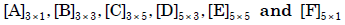are given. Matrices [B] and
[E] are symmetric.
Following statements are made with respect to these matrices.
1. Matrix product [F]T [C]T [B] [C] [F] is a scalar.
2. Matrix product [D]T [F] [D] is always symmetric.
With reference to above statements, which of the following applies?

Detailed Solution for Test: Systems of Linear Equations, Matrix Algebra & Transform Theory- 1 - Question 12

(a)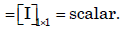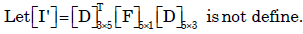Test: Systems of Linear Equations, Matrix Algebra & Transform Theory- 1 - Question 13

The product of matrices (PQ)–1 P is

Detailed Solution for Test: Systems of Linear Equations, Matrix Algebra & Transform Theory- 1 - Question 13

(b)
(PQ) -1 = P Q-1P-1P = Q-1

Test: Systems of Linear Equations, Matrix Algebra & Transform Theory- 1 - Question 14

The matrix A
=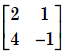is decomposed into a product of a lower triangular matrix [L] and an upper triangular matrix [U]. The properly decomposed [L] and [U] matrices respectively are

Detailed Solution for Test: Systems of Linear Equations, Matrix Algebra & Transform Theory- 1 - Question 14

Ans. (d)

Test: Systems of Linear Equations, Matrix Algebra & Transform Theory- 1 - Question 15

The inverse of the matrix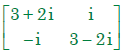is

Detailed Solution for Test: Systems of Linear Equations, Matrix Algebra & Transform Theory- 1 - Question 15

(b)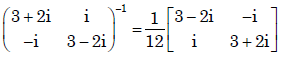Test: Systems of Linear Equations, Matrix Algebra & Transform Theory- 1 - Question 16

The inverse of the 2 × 2 matrix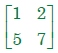is,

Detailed Solution for Test: Systems of Linear Equations, Matrix Algebra & Transform Theory- 1 - Question 16

(a).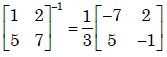Test: Systems of Linear Equations, Matrix Algebra & Transform Theory- 1 - Question 17

There are two containers, with one containing 4 Red and 3 Green balls and the other containing 3 Blue and 4 Green balls. One ball is drawn at random form each container.The probability that one of the ball is Red and the other is Blue will be

Detailed Solution for Test: Systems of Linear Equations, Matrix Algebra & Transform Theory- 1 - Question 17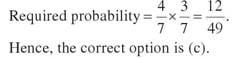Test: Systems of Linear Equations, Matrix Algebra & Transform Theory- 1 - Question 18

The Fourier transform of x(t) = e–at u(–t), where u(t) is the unit step function,

Detailed Solution for Test: Systems of Linear Equations, Matrix Algebra & Transform Theory- 1 - Question 18

Ans. (d)

Test: Systems of Linear Equations, Matrix Algebra & Transform Theory- 1 - Question 19

Given that F(s) is the one-sided Laplace transform of f(t), the Laplace transform of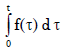is [EC:

Detailed Solution for Test: Systems of Linear Equations, Matrix Algebra & Transform Theory- 1 - Question 19

Ans. (b)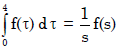……(Lapalace formule)

Test: Systems of Linear Equations, Matrix Algebra & Transform Theory- 1 - Question 20

If f(t) is a finite and continuous function for t, the Laplace transformation is given by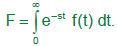For f(t) = cos h mt, the Laplace transformation is…..

## GATE Mechanical (ME) 2023 Mock Test Series

27 docs|243 tests
Information about Test: Systems of Linear Equations, Matrix Algebra & Transform Theory- 1 Page
In this test you can find the Exam questions for Test: Systems of Linear Equations, Matrix Algebra & Transform Theory- 1 solved & explained in the simplest way possible. Besides giving Questions and answers for Test: Systems of Linear Equations, Matrix Algebra & Transform Theory- 1, EduRev gives you an ample number of Online tests for practice

## GATE Mechanical (ME) 2023 Mock Test Series

27 docs|243 tests(Scan QR code)